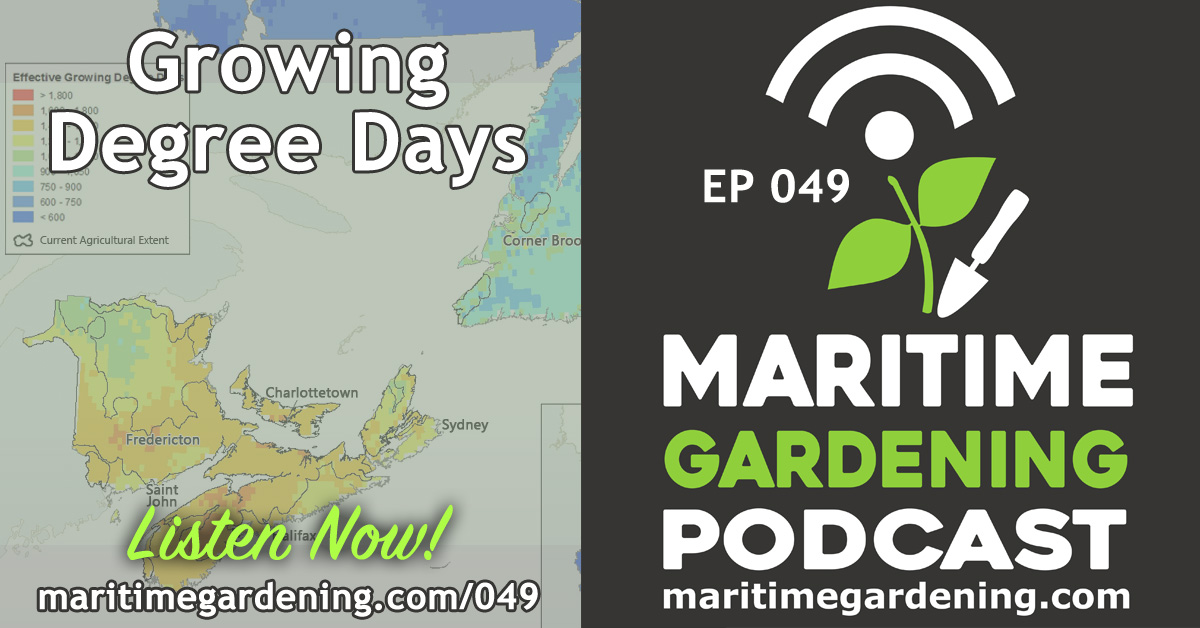#### 048 : Mulling over MulchesOctober 21, 2017

# 049 : Growing Degree Days: A Better Way to Understand your Growing Conditions“Growing Degree Days” (GDDs) are a measure of heat accumulation that indicates how favorable the growing conditions are in your area. If the number is really high, you can grow anything, if it’s low, there’s some things you just can’t grow.

Every plant has a certain number of GDDs it needs to reach maturity.

GDDs tell you what’s possible in your area under normal conditions, or whether you need to cheat to enhance your “normal conditions”.

GDDs are very informative when you move, or are trying to plant something new.

They also enhance your understanding of growth rates and maturity dates, and the conditions that modify them.

How they are calculated:

Conceptual definition:

For a given day,

GDD = average temperature – base temperature

“Average Temperature” means the temperature for a given day, averaged to reflect the high and lows for that day.

“Base Temperature” means a minimum temperature that is required for a given plant species to develop. Some commonly used Base temperatures are 5°C, 10°C, & 13°C.

A crude way to do this is the following

GDD =  ((max temp + min temp) /2 ) – base temp.

Thus, on a day with a high of 20 and a low of 5, given a base temperature of 5°C there would be 7.5 GDDs, and growth.

(20 + 5)/2 -5 = 7.5

On a day with a high of 10 and a low of 5, given a base temperature of 5°C there would be 2.5 GDDs, and thus, less growth.

(10 + 5)/2 – 5 = 2.5

And n a day with a high of 7and a low of 3, given a base temperature of 5°C there would be 0 GDDs, and thus, no growth.

(7 + 3)/2 – 5 =0

Of course, given a base temperature of 13°C, there would be no growth in any of these scenarios.

(20 + 5)/2 -13 = 0

(10 + 5)/2 – 13 = 0

(7 + 3)/2 – 13 = 0

The key value of understanding GDDs is that is allows a far more sophisticated grasp of the growing conditons in your area, what’s possible where you are, and whether you need to cheat to grow certain plants using row tunnels, hoop-houses, coldframes, etc.